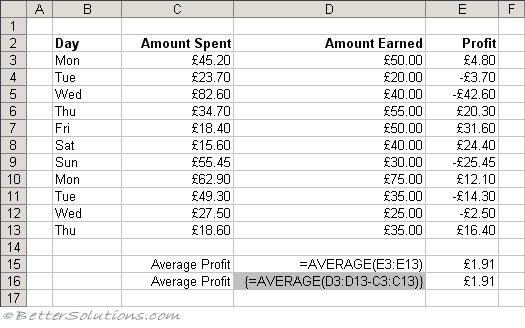# Average cells after subtraction

Lets suppose that we are keeping a record of the amount we spend every day.The formulas in column "E" calculate the difference between the amount earned and the amount spent.
The formula in cell "E3" is just "=D3-C3" and then copied down to cell "E13" and the formula in cell "E15" calculates the average from these values.
You can remove the whole of column "E" from the calculation by using an array formula. This combines calculating the difference and the average all in a single formula.
The formula in cell "E16" first calculates the difference and then returns an array of differences to the AVERAGE function.